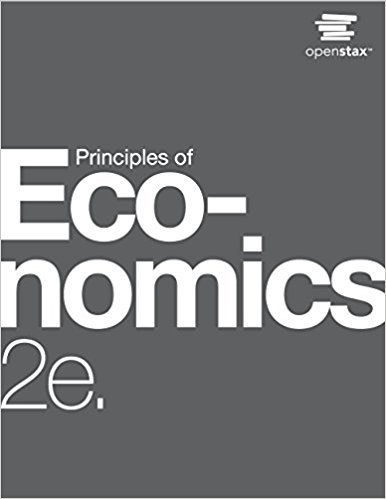×
Get Full Access to Principles Of Economics - 2 Edition - Chapter 24 - Problem 4
Get Full Access to Principles Of Economics - 2 Edition - Chapter 24 - Problem 4

×

# Solved: In the AD/AS model, what prevents the economy from achieving equilibrium atISBN: 9781947172364 471

## Solution for problem 4 Chapter 24

Principles of Economics | 2nd Edition

• Textbook Solutions
• 2901 Step-by-step solutions solved by professors and subject experts
• Get 24/7 help from StudySoup virtual teaching assistantsPrinciples of Economics | 2nd Edition

4 5 1 270 Reviews
24
4
Problem 4

In the AD/AS model, what prevents the economy from achieving equilibrium at potential output?

Step-by-Step Solution:
Step 1 of 3

Multicollinearity Multicollinearity When your (independent) variables correlate highly with each other (as they are measuring the same thing) High collinearity increases the likelihood of a type 2 error (so significant difference found when one exists) Multicollinearity Multicollinearity Reduce likelihood of Type 2 error To reduce Multicollinearity can combine highly correlated variables or reduce the number of variables check for multicollinearity What is the 5th step in the model building process multicollinearity What is a condition that occurs when independent variables are correlated with one another collinearity or intercorrelation What are two other names for multicollinearity multicollinearity What exists in virtually all multiple regression models only when two or

Step 2 of 3

Step 3 of 3

##### ISBN: 9781947172364

Unlock Textbook Solution